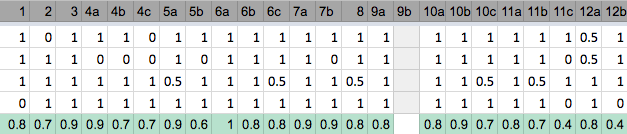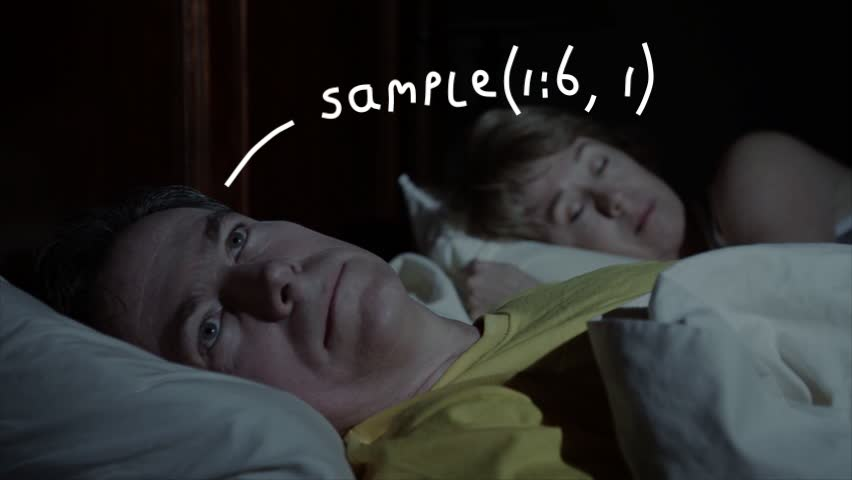October 6, 2017

## Assignments

• Compare mistakes with answer sheet
• Remaining questions during tutorial
•• 11c: What is the probability of obtaining any of the numbers 2, 4, or 6 if a die is tossed three times in a row?
• Not tossing a 2, 4, or 6 in 3 tosses is \(.5^3\). Tossing 2, 4, or 6 at least once is \(1-(.5^3)\).
• Or \(.5+.5^2+.5^3\)